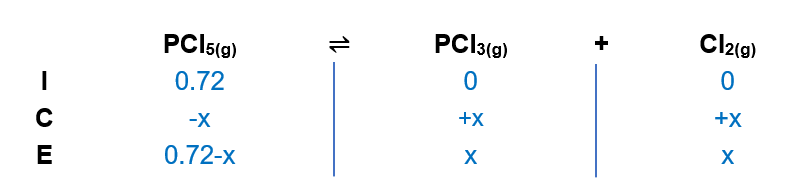# Problem: A 0.72-mol sample of PCl 5 is put into a 1.00-L vessel and heated. At equilibrium, the vessel contains 0.40 mol of PCl3(g) and 0.40 mol of Cl2(g). Calculate the value of the equilibrium constant for the decomposition of PCl5 to PCl3 and Cl2 at this temperature.

###### FREE Expert Solution

We are being asked to calculate the equilibrium constant, Kc for the given equilibrium reaction:

PCl5 (g)  Cl2 (g) + PCl(g)

When dealing with equilibrium and Kp:

Kc → equilibrium units are in molarity
Kc is an equilibrium expression:

$\overline{){{\mathbf{K}}}_{{\mathbf{c}}}{\mathbf{=}}\frac{\mathbf{products}}{\mathbf{reactants}}}$

only aqueous and gaseous species are included in the equilibrium expression
▪ the coefficient of each compound in the reaction equation will be the exponent of the concentrations in the equilibrium expression

Given:

Initial Concentrations:

[PCl5] = 0.72 mol / 1L = 0.72 M

In order to calculate the equilibrium concentrations of species, we need to use an ICE ChartSince x is equal to 0.40 M, the concentration of PCl5 at equilibrium is:

94% (228 ratings)###### Problem Details

A 0.72-mol sample of PCl 5 is put into a 1.00-L vessel and heated. At equilibrium, the vessel contains 0.40 mol of PCl3(g) and 0.40 mol of Cl2(g). Calculate the value of the equilibrium constant for the decomposition of PCl5 to PCl3 and Cl2 at this temperature.

Frequently Asked Questions

What scientific concept do you need to know in order to solve this problem?

Our tutors have indicated that to solve this problem you will need to apply the ICE Chart concept. You can view video lessons to learn ICE Chart. Or if you need more ICE Chart practice, you can also practice ICE Chart practice problems.

What professor is this problem relevant for?

Based on our data, we think this problem is relevant for Professor Selampinar's class at UCONN.

What textbook is this problem found in?

Our data indicates that this problem or a close variation was asked in Chemistry - OpenStax 2015th Edition. You can also practice Chemistry - OpenStax 2015th Edition practice problems.